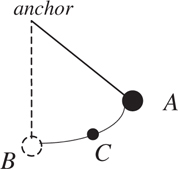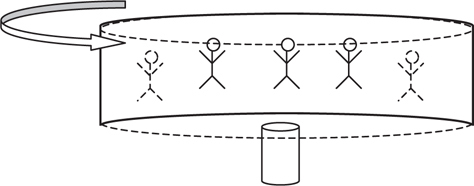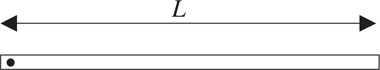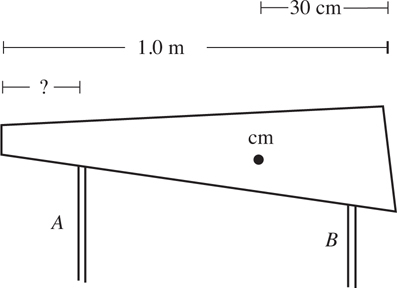# AP Physics C: Mechanics Practice Test 10

### Test Information11 questions15 minutes

1.A ball of mass m anchored to a string swings back and forth to a maximum position A, as shown above. Point C is partway back to the vertical position. What is the direction of the mass’s acceleration at point C?

2.In a carnival ride, people of mass m are whirled in a horizontal circle by a floorless cylindrical room of radius r, as shown in the diagram above. If the coefficient of friction between the people and the tube surface is μ, what minimum speed is necessary to keep the people from sliding down the walls?

3.The uniform, rigid rod of mass m, length L, and rotational inertia I shown above is pivoted at its left-hand end. The rod is released from rest from a horizontal position.

What is the linear acceleration of the rod’s center of mass the moment after the rod is released?

4.The uniform, rigid rod of mass m, length L, and rotational inertia I shown above is pivoted at its left-hand end. The rod is released from rest from a horizontal position.

What is the linear speed of the rod’s center of mass when the mass passes through a vertical position?

5.The 1.0-m-long nonuniform plank, shown above, has weight 1000 N. It is to be supported by two rods, A and B, as shown above. The center of mass of the plank is 30 cm from the right edge. Each support bears half the weight of the plank. If support B is 10 cm from the right-hand edge, how far from the left-hand edge should support A be?

6. A mass m on a spring oscillates on a horizontal surface with period T. The total mechanical energy contained in this oscillation is E. Imagine that instead a new mass 4m oscillates on the same spring with the same amplitude. What is the new period and total mechanical energy?

7. A mass m is attached to a horizontal spring of spring constant k. The spring oscillates in simple harmonic motion with amplitude A. What is the maximum speed of this simple harmonic oscillator?

8. An empty bottle goes up and down on the surface of the ocean, obeying the position function x = Acos(ωt). How much time does this bottle take to travel once from its lowest position to its highest position?

9. The Space Shuttle orbits 300 km above the Earth’s surface; the Earth’s radius is 6400 km. What is the acceleration due to Earth’s gravity experienced by the Space Shuttle?

10. An artificial satellite orbits Earth just above the atmosphere in a circle with constant speed. A small meteor collides with the satellite at point P in its orbit, increasing its speed by 1%, but not changing the instantaneous direction of the satellite’s velocity. Which of the following describes the satellite’s new orbit?

11. Mercury orbits the sun in about one-fifth of an Earth year. If 1 AU is defined as the distance from the Earth to the sun, what is the approximate distance between Mercury and the sun?